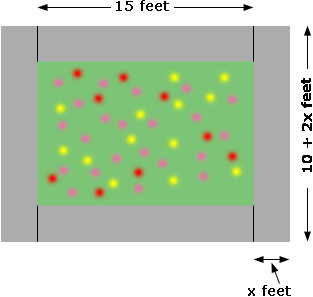SEARCH HOMEMath Central Quandaries & QueriesQuestion from Barbara, a student: A rectangular flower bed measuring 10 feet by 15 feet has a surrounding walkway on all sides that measure 186 square feet. How wide is the walkway?Barbara,

You should draw a diagram. I assumed the walkway was the same width all the way around and called the width x feet. I then cut the walkway into 4 pieces as in the diagram.Two of the pieces are 15 feet by x feet and the other two are 10 + 2x feet by x feet. What is the total area of the four pieces? Set this equal to 186 square feet and solve for x.

HarleyMath Central is supported by the University of Regina and The Pacific Institute for the Mathematical Sciences.